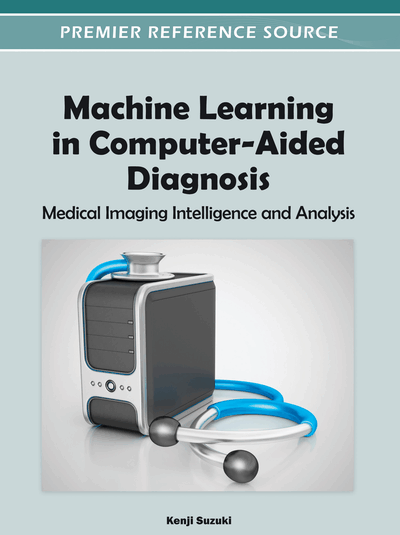Manifold Learning for Medical Image Registration, Segmentation, and Classification

Paul Aljabar (Imperial College London, UK), Robin Wolz (Imperial College London, UK) and Daniel Rueckert (Imperial College London, UK)
DOI: 10.4018/978-1-4666-0059-1.ch017

Abstract

The term manifold learning encompasses a class of machine learning techniques that convert data from a high to lower dimensional representation while respecting the intrinsic geometry of the data. The intuition underlying the use of manifold learning in the context of image analysis is that, while each image may be viewed as a single point in a very high-dimensional space, a set of such points for a population of images may be well represented by a sub-manifold of the space that is likely to be non-linear and of a significantly lower dimension. Recently, manifold learning techniques have begun to be applied to the field of medical image analysis. This chapter will review the most popular manifold learning techniques such as Multi-Dimensional Scaling (MDS), Isomap, Local linear embedding, and Laplacian eigenmaps. It will also demonstrate how these techniques can be used for image registration, segmentation, and biomarker discovery from medical images.
Chapter Preview
Top

Manifold Learning Techniques

This section describes some of the most important manifold learning techniques. It follows the comprehensive overviews given in (van der Maaten, 2009) and (Pless, et al., 2009). A set of images {x1,...,xN} is described by N images, each being defined as a vector of intensities, where D is the number of voxels per image or region of interest. Assuming that {x1,...,xN} lie on or near a d dimensional manifoldembedded inand, it is possible to learn a new, low dimensional representationwithof the input images.

Complete Chapter List

Search this Book:
Reset